# Initialize, Reset, and Terminate State of Simulink Block

This example shows how to use the Initialize Function block and Reset Function block to initialize and reset the Discrete-Time Integrator block states and how to use the Terminate Function block to terminate the integration process based on an event schedule.

### Model Using Initialize Function, Reset Function, and Terminate Function Block

The `ref_IRTFunctionBlocks` model uses one Initialize Function block, two Reset Function blocks, and one Terminate Function block. The two Reset Function blocks reset the two Discrete-Time Integrator block states.

In this example, the State Writer blocks inside the Initialize and Reset Function blocks set the states of the Discrete-Time Integrator blocks. The State Reader block inside the Terminate Function block reads the states of the Discrete-Time Integrator blocks. For more information about the blocks that allow State Writer and State Reader blocks, see State Writer and State Reader.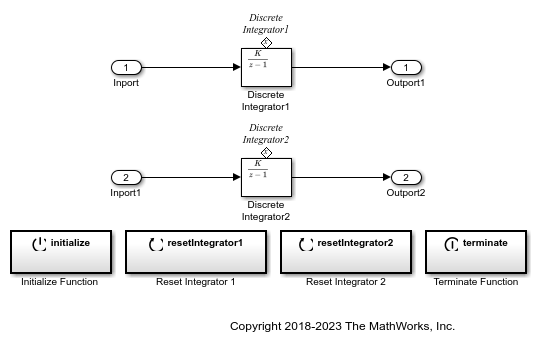• The Initialize Function block uses the State Writer block to set the Discrete-Time Integrator block states when the Initialize Function block receives a initialize function-call event.• The Reset Function block uses the State Writer block to reset the Discrete-Time Integrator block states when the Reset Function blocks receive the reset function-call events.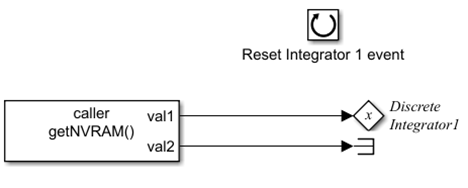Two Reset Function blocks are used at the root level of the `ref_IRTFunctionBlocks` model. When using multiple Reset Function blocks at the same model hierarchy level, you must use unique event names for the Event name parameter of the Event Listener blocks for those Reset Function blocks. In this model, Event name is `resetIntegrator1` for the `Reset Integrator 1` block, and `resetIntegrator2` for the `Reset Integrator 2` block.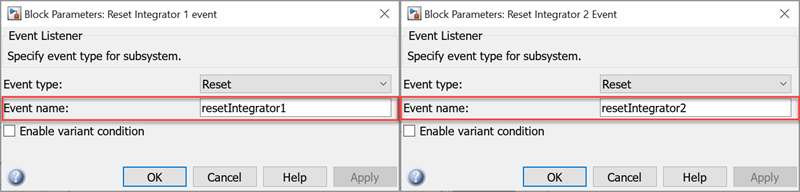• The Terminate Function block terminates the integration when the block receives a terminate function-call event.### Model Behavior

The model is simulated based on function-call events and uses the Discrete-Time Integrator block state values from the data store.

• The `ref_IRTFunctionBlocks` model is referenced by a Model block at the root level of the `ex_IRTFunctionBlocks` model.

• The event scheduler is modeled using Stateflow® charts that generate function-call events. The model executes based on the function-call events.

• `Discrete Integrator1` and `Discrete Integrator2` blocks receive the input from the Constant blocks named `Input 1` and `Input 2` respectively.

• The Initialize Function block and the Reset Function blocks read the Discrete-Time Integrator block states from the data store `NVRAM`. The Terminate Function block writes the Discrete-Time Integrator block states to the data store.### Model Function-Call Events Using Event Scheduler

A Stateflow® chart is used to model an event schedule that generates the initialize, reset, and terminate function-call events. These function-call events control the execution of the `ex_IRTFunctionBlocks` model. For information about modeling the event scheduler and connecting the chart to the Model block, see Create Test Harness to Generate Function Calls.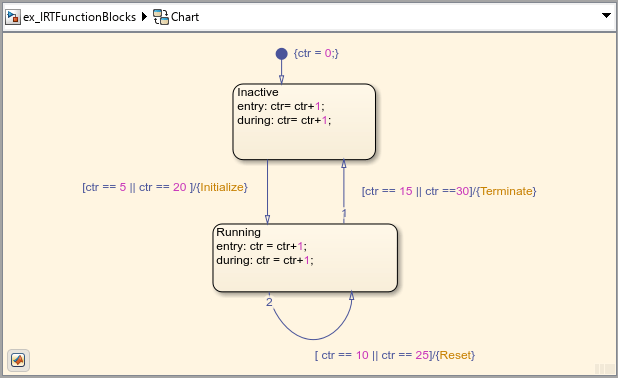### Use Data Store to Model Nonvolatile Memory

In this example, a data store is used to model a nonvolatile memory that stores Discrete-Time Integrator block state values at the root level of the `ex_IRTFunctionBlocks` model. For more information, see Data Store Basics.

The data store is created using a Data Store Memory block. A Data Store Read block inside the `getNVRAM` function and a Data Store Write block inside the `setNVRAM` function read and write the states of the Discrete-Time Integrator blocks respectively from the data store. For more information about creating data store, see Model Global Data by Creating Data Stores. This data store uses `Simulink.Bus` `NVRAM` that consists of `Simulink.BusElement` objects named `s1` and `s2`. `s1` corresponds to the `Discrete Integrator1` block, and `s2` corresponds to the `Discrete Integrator2` block. For more information, see Data Store Memory, Data Store Read and Data Store Write.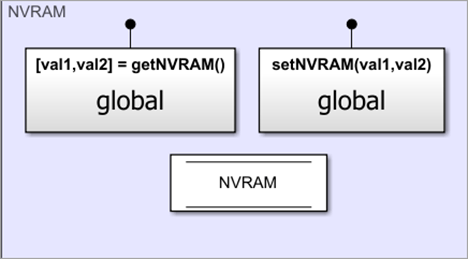• Initial value of `s1` is set to 5. The initial value of `s2` is set to -5.• The function `getNVRAM` uses the Data Store Read block to read the data from the Data Store Memory block. The State Writer block inside the Initialize Function and Reset Function blocks obtain the data from the data store and write to the Discrete-Time Integrator block states.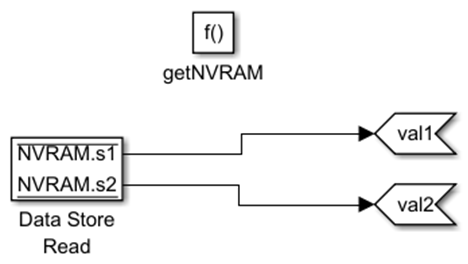• The State Reader block inside the Terminate Function block reads the Discrete-Time Integrator block state values. The function `setNVRAM` uses the Data Store Writer block to write the data to the Data Store Memory block.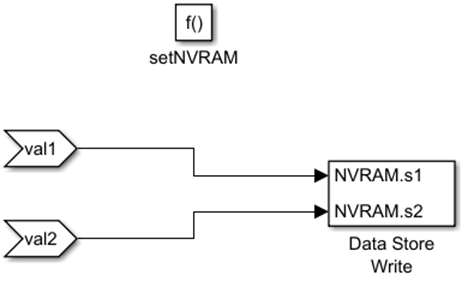### Results

The model executes based on the function-call events. The Discrete-Time Integrator block uses forward Euler accumulation integration method. For more information about the integration method, see Discrete-Time Integrator.

You can use the Scope block to view the output of both the Discrete-Time Integrator blocks at different time steps. The first row ( `channel1` ) and second row ( `channel2` ) of the Scope block represent the output of the `Discrete Integrator1` and `Discrete Integrator2` blocks respectively.

From the Scope block output, you can observe the following:

• At time step 5, the Initialize Function block initializes the state of the `Discrete Integrator1` block to 5 and the `Discrete Integrator2` block to -5.

• At time step 10, the Reset Function block resets the state of the `Discrete Integrator1` block to 5 while the `Discrete Integrator2` block to -5.

• At time step 15, the Terminate Function block terminates the integration process for both the Discrete-Time Integrator blocks. The state value of both the blocks computed at time step 14 (15 for the `Discrete Integrator1` block and -15 for the `Discrete Integrator2` block) are written to the Data Store Memory block. The output computed at time step 14 represents the output from time step 15 through time step 19.

• At time step 20, the Initialize Function block is retriggered, and the states of both the Discrete-Time Integrator blocks are initialized to the state values stored in step 3.

• At time step 25, the Reset Function block resets the states of both the Discrete-Time Integrator blocks to the state values stored in step 3.

• At time step 30, the Terminate Function block terminates the integration processes of both the Discrete-Time Integrator blocks, and the states computed at time step 29 are written to the Data Store Memory block.